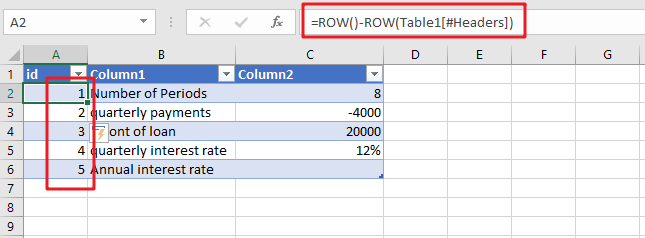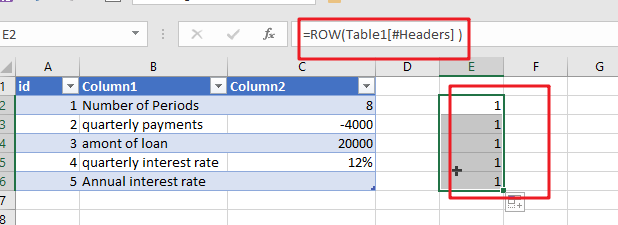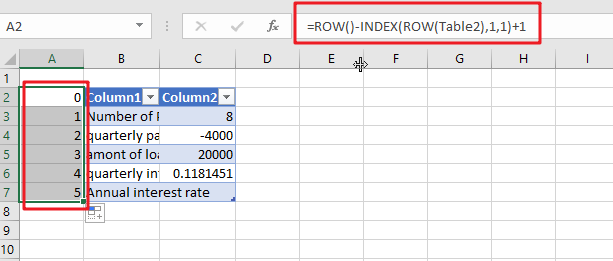# Creating a Table with Automatic Row NumberingWhen creating an Excel Table, you may use a calculation relying on the ROW function to insert row numbers automatically. The formula in A2, which has been copied down, is as follows:

`=ROW()-ROW(Table1[#Headers])`

Note: the default table name is Table1. And #Headers is the header in the Table1.

## Let’s See How This Formula Works:

When there is no parameter, the ROW function will return the row number for the current row. If A cell reference is supplied, and ROW function will retrieve the number of rows of the selected cell in question. When a range is specified, ROW returns the row number corresponding to the first row in the range.

For the above formula, there is no argument supplied to ROW, the first ROW function returns 2, located in cell A2. The second ROW function makes use of a structured reference, as follows:

`=ROW(Table1[#Headers])`The formula above works well as long as there is a header row in the table; however, the formula will fail if the heading row is disabled. If you are dealing with a table that does not have a header row, you may make use of the following method:

`=ROW()-INDEX(ROW(Table2),1,1)+1`

As in the previous formula, the initial ROW function will return the row that is currently being shown. Using the INDEX function, the first cell in the range Table2 (cell B2) is passed on to the second-ROW feature, which always returns the number 5. The following is how the formula works for the first three rows of the table:Regardless of whether or not the header row is enabled, this equation will continue to operate correctly.

### Related Functions

• Excel INDEX function
The Excel INDEX function returns a value from a table based on the index (row number and column number)The INDEX function is a build-in function in Microsoft Excel and it is categorized as a Lookup and Reference Function.The syntax of the INDEX function is as below:= INDEX (array, row_num,[column_num])…
• Excel ROW function
The Excel ROW function returns the row number of a cell reference.The ROW function is a build-in function in Microsoft Excel and it is categorized as a Lookup and Reference Function.The syntax of the ROW function is as below:= ROW ([reference])….